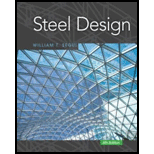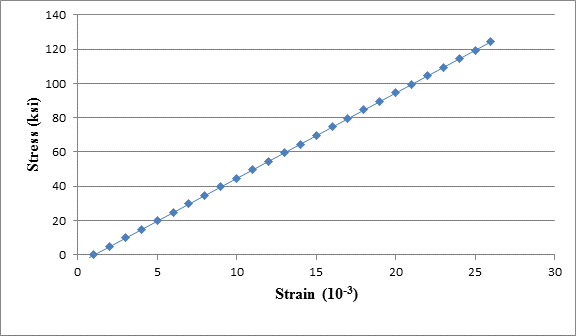Chapter 1, Problem 1.5.6P### Steel Design (Activate Learning wi...

6th Edition
Segui + 1 other
ISBN: 9781337094740

#### Solutions

Chapter
Section### Steel Design (Activate Learning wi...

6th Edition
Segui + 1 other
ISBN: 9781337094740
Textbook Problem

# The data in Table 1.5.3 were obtained from a tensile test of a metal specimen with a rectangular cross section of 0. 2 0 11 in . 2 in area and a gage length (the length over which the elongation is measured) of 2.000 inches. The specimen was not loaded to failure.a. Generate a table of stress and strain values.b. Plot these values and draw a best-fit line to obtain a stress-strain curve.c. Determine the modulus of elasticity from the slope of the linear portion of the curve.d. Estimate the value of the proportional limit.e. Use the 0. 2 % offset method to determine the yield stress.To determine

(a)

The stress and strain values.

Explanation

Given:

The cross-sectional area is 0.2011in2.

The gage length is 2inches.

Concept Used:

Write the equation to calculate tensile stress.

f=PA      ...... (I)

Here, tensile stress is f, load is P and the cross-sectional area is A.

Write the equation to calculate strain.

ε=eL      ...... (II)

Here, the elongation is e and the gage length is L and the strain is ε.

Calculate the stress and strain values by substituting corresponding elongation and load values in the Equation (I) and (II).

The following table shows the stress and strain values.

 Load (kips) Elongation (×10−3 in) Stress f=PA Stress (ksi) Strain ε=eL Strain (×10−3) 0 0 f=00.2011 0 ε=02 0 1 0.160 f=10.2011 4.97265 ε=0.16×1032 0.08 2 0.352 f=20.2011 9.9453 ε=0.352×1032 0.176 3 0.706 f=30.2011 14.918 ε=0.706×1032 0.353 4 1.012 f=40.2011 19.8906 ε=1.012×1032 0.506 5 1.434 f=50.2011 24.8633 ε=1.434×1032 0.717 6 1.712 f=60.2011 29.8359 ε=1.712×1032 0.856 7 1.986 f=70.2011 34.8086 ε=1.986×1032 0.993 8 2.286 f=80.2011 39.7812 ε=2.286×1032 1.143 9 2.612 f=90.2011 44.7539 ε=2.612×1032 1.306 10 2.938 f=100.2011 49.7265 ε=2.938×1032 1.469 11 3.274 f=110.2011 54.6992 ε=3.274×1032 1.637 12 3.632 f=120.2011 59.6718 ε=3.632×1032 1.816 13 3.976 f=130.2011 64.6445 ε=3.976×1032 1.988 14 4.386 f=140.2011 69.6171 ε=4.386×1032 2.193 15 4.640 f=150.2011 74.5898 ε=4.64×1032 2.32 16 4.988 f=160.2011 79.5624 ε=4.988×1032 2.494 17 5.432 f=170.2011 84.5351 ε=5.432×1032 2
To determine

(b)

A plot of the stress-strain curve.

To determine

(c)

The modulus of elasticity from the slope of linear portion of curve.

To determine

(d)

The value of proportional limit.

To determine

(e)

The yield stress in the metal specimen.

### Still sussing out bartleby?

Check out a sample textbook solution.

See a sample solution

#### The Solution to Your Study Problems

Bartleby provides explanations to thousands of textbook problems written by our experts, many with advanced degrees!

Get Started

## Additional Engineering Solutions

#### Find more solutions based on key concepts